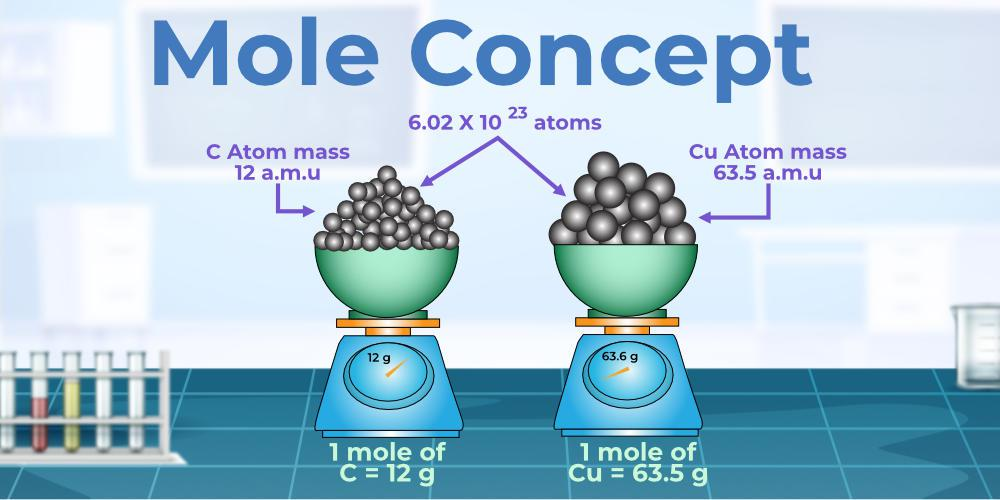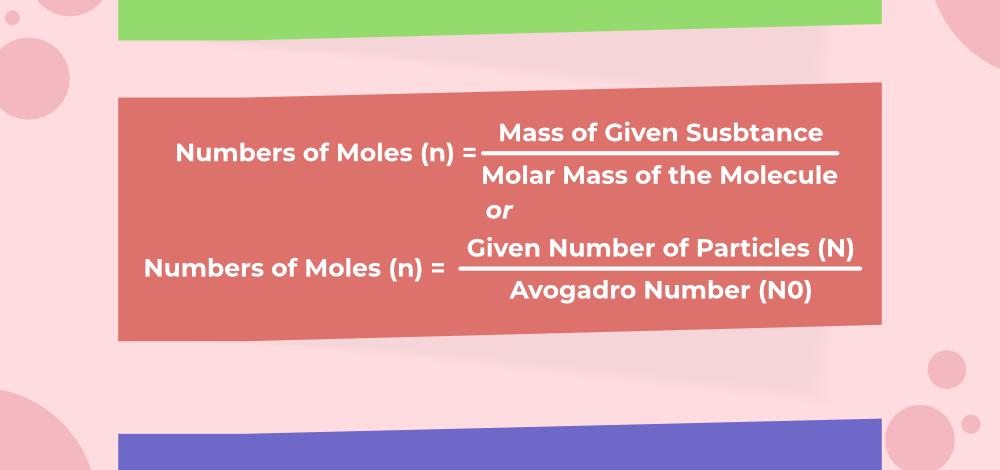Open in App
Not now

# Molecular Mass

• Last Updated : 19 Mar, 2023

Molecular Mass is the mass of all constituents of an atom such as electrons, protons, and neutrons. In ancient India and Greece, philosophers have first given the idea of atoms and deeply studied them. Around 500 BC.  Maharishi Kanad, an Indian philosopher states that if we divide the “Padarth” (matter), we get small-small particles. Lastly, the further division of particles was not possible, he named that particle a “Parmanu”.

Around the same era, ancient  Democritus and Leucippus, Greek philosophers – suggested that if we go on the dividing matter, a stage will come when particles obtained cannot be further divisible. they called these indivisible particles atoms (meaning indivisible). All this was just the theory, not a practical or experimental consideration. By the end of the 18th century, scientists search more and get the difference between compounds and elements, and that created interest in finding out how and why they combine and what happens after that. Antoine L. Lavoisier further studies more on laws of chemical combinations.

Everything around is made up of very small units these units are atoms in the language of science, very small in the sense is in the range of 0.1 – 0.5 nanometers.

## What is a Molecule?

A molecule is a group of two or more atoms that are chemically bonded together, i.e, tightly held together by attractive forces. A molecule can be defined as the tiniest particle of an element or a compound that is fit for a free existence and shows all the properties of its parent substance.

Atoms of the same or of different elements can form molecules. Following is the list of some important molecules with their combining ratios and mass ratios as:

## Mole Concept

It is easier to use the quantity of substance in a number of molecules or atoms instead of their mass, so the unit called Mole is used.If the element is weighed and it is equal to its atomic weight in grams, then it contains a certain amount of element, which represents one mole of an element, and that certain amount of element is 6.022 x 1023 atoms of an element. 1 mole of any molecule or atom is equal to its atomic mass or molecular mass in grams. The number of particles in 1 mole = 6.022 x 1023 atoms or molecules and this is known as the Avogadro number.

The number of particles in 1 mole:

• 1 mole of hydrogen atoms shows 6.022 × 1023 hydrogen atoms.
• 1 mole of hydrogen molecules shows 6.022 × 1023 hydrogen molecules.
• 1 mole of water molecules shows 6.022 × 1023 water molecules.

Mathematically, the number of moles is defined as:Example 1: Find the number of moles present inside the 24.088 ×1023 particles of water.

Given that,

The number of particles present, N = 24.088 ×1023

The number of moles (n) = Number of particles (N) / Avogadro number (N0)

= 24.088 ×1023 / 6.022 x 1023

= 4 moles

## What is Molecular mass?

The molecular mass of an element is defined as the sum of all the masses of the elements present inside the molecule. e.g. the molecular mass of water, in which there are two hydrogen atoms and one oxygen atom present, is 18 (i.e. 2 + 16). Also called its molecular weight.

### Unit of Molecular Mass

SI unit of Molecular Mass or Molecular Weight is an atomic mass unit which in short is called a.m.u. It is sometimes represented by u which represents unified mass.

## Molecular Mass of some Elements

Molecular mass is predicted by multiplying the number of atoms with the atomic mass of an element in the molecule and then adding the masses of all the elements in the molecule. The following table shows the atomic mass of the first twenty elements of the periodic table as,

### What are Ions?

It is an electrically charged species or atom or group of atoms. It is formed by either gaining or losing one or more electrons by an atom.

Ions are of two types:

Cation

Cations are formed by the loss of one or more electrons from an atom, so they are positively charged ions. e.g.

• On losing one electron sodium atom forms a sodium ion Na as,

Na – e−  →  Na+

• On losing two electrons Magnesium forms a magnesium ion as,

Mg – e → Mg+

Again it loses one more electron to complete its octet as,

Mg+ – e–  → Mg2+

Anion

It is a negatively charged ion and is formed by the gain of one or more electrons by an atom. e.g.

• On gaining one electron chlorine form a chloride ion Cl as,

Cl + e  →  Cl

• On gaining two electrons oxygen form an oxide ion as,

O  +  e–  → O–

Again it gains one more electron to complete its octet as,

O + e- →  O2-

## Calculation of Molecular Mass

Molecular mass is predicted by multiplying the number of atoms with the atomic mass of an element in the molecule and then adding the masses of all the elements in the molecule.

1. Make sure to analyze the chemical formula to determine the number of atoms of each element in the compound.
2. Multiply the number of atoms present in the compound by the atomic weight of each element.
3. Add up all the mass and assign its unit as grams/mole.

Example 1: Determine the Molar mass of CO2.

First Calculate the number of each atom, and assign their atomic weight individually

The number of carbon atoms × its atomic mass: 1 × 12 = 12

The number of oxygen atoms × its atomic mass: 2 × 12 = 24

Then add the values i.e, 12 + 24= 36

Therefore the molar mass of CO2 is 36 g/mol.

Example 2: Determine the formula unit mass of CaCl2.

The formula unit mass of CaCl2 = Atomic mass of Ca + 2 × (Atomic mass of Cl)

= 40 + 2 × 35.5

= 40 + 71

= 111 a.m.u.

## Solved Examples of Molecular Mass

Example 1: What is an Atomicity? List the atomicity of different main elements of the periodic table.

Atomicity is defined as the number of atoms present in one molecule of an element or a compound is known as its atomicity. below is the table that shows the atomicity.

Example 2: What is the molar mass of sodium carbonate, Na2CO3?

Since, a molecule of sodium carbonate contains two atoms of sodium (Na), one atom of carbon (C) and three atoms of oxygen (O). The molecular weight would be

Na: 2 × 23.0 g =  46 g

C: 1 × 12.0 g = 12 g

O: 3 × 16 g = 48 g

When we add up the total values i.e, 46 g + 12 g + 48 g = 106 g

Hence, the molar mass of Na2CO3 is 106 g/mole.

Example 3: Calculate the molar mass of H2SO4.

The molar mass can be calculated as:

Molar mass of H2SO4  = (2 × Mass of Hydrogen) + (1 × Mass of Sulphur) + (4 × Mass of Oxygen)

=  (2 × 1 + 1 × 32 + 4 × 16) g/mol

= (2 + 32 + 64) g/mol

= 98 g/mol

Example 4: State the number of hydrogen (H) atoms in 1 g of Hydrogen.

Since one gram of Hydrogen (H) contains 1 mole of Hydrogen which is equal to 6.022 x 1023 atoms.

Example 5: How many atoms are present in:

(a) H2S molecule and

(b) PO4−3 ion?

(a) In H2S, there is one sulphur and two hydrogen atoms present so a total of 3 atoms are present.

(b) In PO4−3, one Phosphorus and 4 oxygen atoms are present so a total of 5 atoms are present.

Example 6: What is the mass of:

(a) 1 mole of nitrogen atoms?

(b) 4 moles of aluminium (Al) atoms (Atomic mass Al= 27)?

(c) 20 moles of sodium sulphite (Na2SO3)?

(a) 1 mole of Nitrogen = 1 × Atomic mass of nitrogen atom

= 1 × 14 g

= 14 g

(b) 4 moles of aluminium atoms = 4 × Atomic mass of aluminium atom

= 4 × 27 g

= 108 g

(c) 20 moles of sodium sulphite (Na2SO3) = 20 (2 × Atomic mass of Na + 1 × Atomic mass of sulphur + 3 × Atomic mass of oxygen)

= 20 (2 × 23 g+1 × 32 g + 3 × 16 g)

= 20 (46 g + 32 g + 48 g)

= 20 × 126 g

= 2520 g

## FAQs on Molecular Mass

### Question 1: What is molecular mass?

The molecular mass of an element is defined as the sum of all the masses of the elements present inside the molecule. e.g. the molecular mass of water, in which there are two hydrogen atoms and one oxygen atom present, is 18 (i.e. 2 + 16).

### Question 2: What is the molecular mass of glucose?

Chemical formula for glucose is C6H12O6

Thus, molecular mass of glucose = 6×12 + 12×1 + 6×16 = 72 + 12 + 96 = 180

### Question 3: What is the molecular mass of oxygen?

Molecular Mass of Oxygen is aprroxiamately 16 gm/mole.